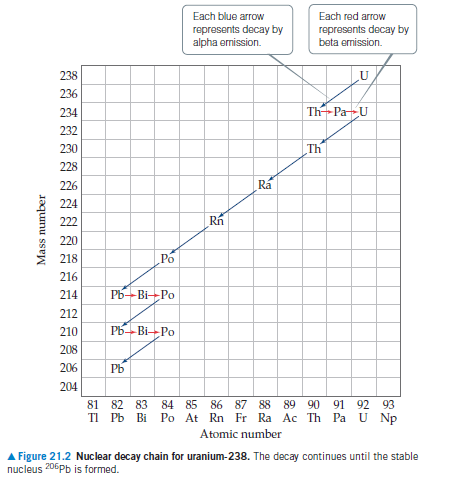# Problem: Indicate whether each of the following nuclides lies within the belt of stability in Figure 21.2 in the textbook.(a) neon-24,(b) chlorine-32, (c) tin-108, (d) polonium-216. For any that do not, describe a nuclear decay process that would alter the neutron-to-proton ratio in the direction of increased stability.

###### FREE Expert Solution
100% (369 ratings)
###### Problem Details

Indicate whether each of the following nuclides lies within the belt of stability in Figure 21.2 in the textbook.

(a) neon-24,
(b) chlorine-32,
(c) tin-108,
(d) polonium-216.

For any that do not, describe a nuclear decay process that would alter the neutron-to-proton ratio in the direction of increased stability.Frequently Asked Questions

What scientific concept do you need to know in order to solve this problem?

Our tutors have indicated that to solve this problem you will need to apply the Band of Stability concept. You can view video lessons to learn Band of Stability. Or if you need more Band of Stability practice, you can also practice Band of Stability practice problems.